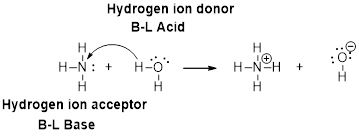# Consider the following ions: NH 4 + , CO 3 2− , Br − , S 2− , and ClO 4 − . (a) Which of these ions in water gives an acidic solution and which gives a basic solution? (b) Which of these anions will have no effect on the pH of an aqueous solution? (c) Which ion is the strong base? (d) Write a chemical equation for the reaction of each basic anion with water.### Chemistry & Chemical Reactivity

9th Edition
John C. Kotz + 3 others
Publisher: Cengage Learning
ISBN: 9781133949640

#### Solutions

Chapter
Section### Chemistry & Chemical Reactivity

9th Edition
John C. Kotz + 3 others
Publisher: Cengage Learning
ISBN: 9781133949640
Chapter 16, Problem 84GQ
Textbook Problem
74 views

## Consider the following ions: NH4+, CO32−, Br−, S2−, and ClO4−. (a) Which of these ions in water gives an acidic solution and which gives a basic solution? (b) Which of these anions will have no effect on the pH of an aqueous solution? (c) Which ion is the strong base? (d) Write a chemical equation for the reaction of each basic anion with water.

(a)

Interpretation Introduction

Interpretation:

The given ions (NH4+, CO32-, Br-, S2- and ClO4-) in water which gives an acidic solution and a basic solution has to be determined.

Concept Introduction:

The pH of a solution is negative logarithm of the hydrogen ion concentration.

pH = -log[H+]

The pH of the solution below 7, then the solution acidic. If the pH of the solution is above 7 then the solution basic.The pH of the solution is equal to the 7 ,then the solution is neutral.

Salt anions may accept hydrogen ions from the water producing a basic solution.

Salt cations may donate hydrogen ions to the water producing an acidic solution.

### Explanation of Solution

NH4++H2OH3O++NH3

In the above reaction NH4+ salt cation donate hydrogen ions to the water producing an acidic solution.

CO32-:

CO32-+H2OHCO3-+OH-

In the above reaction, salt anions CO32- accept hydrogen ions from the water producing a basic solution.

Br-:

Br-+H2OHBr+OH-

In the above reaction, Br- salt anions accept hydrogen ions from the water producing a basic solution

(b)

Interpretation Introduction

Interpretation:

The anions (NH4+, CO32-, Br-, S2- and ClO4-) will have no effect on the pH of an aqueous solution has to be determined.

Concept Introduction:

The pH of a solution is negative logarithm of the hydrogen ion concentration.

pH = -log[H+]

The pH of the solution below 7, then the solution acidic. If the pH of the solution is above 7 then the solution basic.The pH of the solution is equal to the 7 ,then the solution is neutral.

Salts derived from the strong acids and strong bases. These are consists of cations from strong bases and anions from the strong acids. These have no effect on the pH when dissolved in water.

(c)

Interpretation Introduction

Interpretation:

The strong base has to be identified.

Concept Introduction:

Bronsted –Lowry definitions:

A Bronsted –Lowry acid is a proton donor, it donates a hydrogen ion, H+

A Bronsted-Lowry base is a proton acceptor, it accepts a hydrogen ion H+.Strong bases are completely ionized in water. According to Brosted ,strong base accepts proton.

(d)

Interpretation Introduction

Interpretation:

The chemical equation for the reaction of each basic anion with water has to be written.

Concept Introduction:

The pH of a solution is negative logarithm of the hydrogen ion concentration.

pH = -log[H+]

The pH of the solution below 7, then the solution acidic. If the pH of the solution is above 7 then the solution basic. The pH of the solution is equal to the 7 ,then the solution is neutral.

Basic anions react with water to form their respective molecules.

### Still sussing out bartleby?

Check out a sample textbook solution.

See a sample solution

#### The Solution to Your Study Problems

Bartleby provides explanations to thousands of textbook problems written by our experts, many with advanced degrees!

Get Started